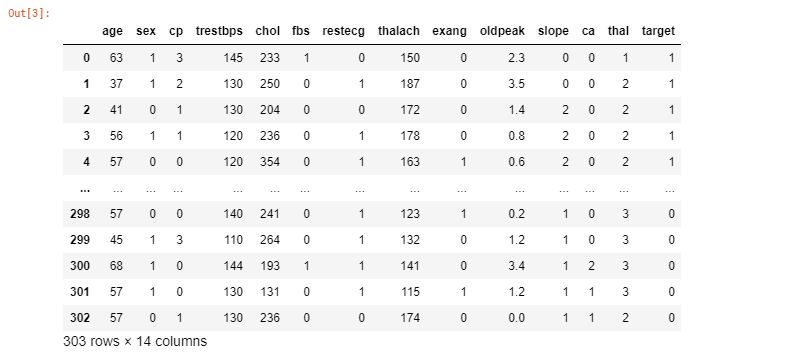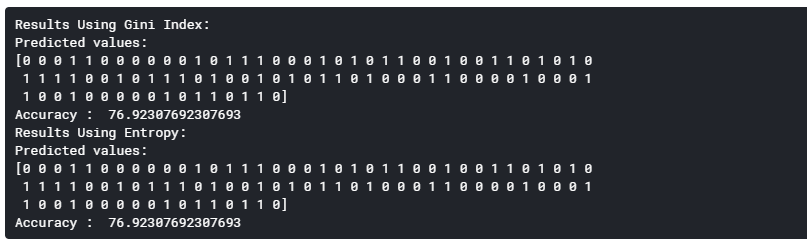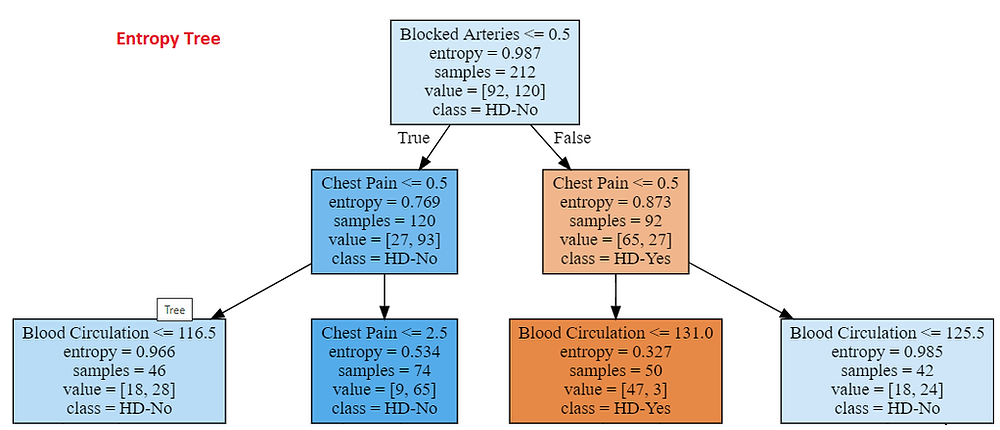Search

# Detection of heart disease using Decision Tree ClassifierDecision Tree is one of the most popular and powerful classification algorithms in machine learning, that is mostly used for predicting categorical data. Entropy/Information Gain and Gini Impurity are 2 key metrics used in determining the relevance of decision making when constructing a decision tree model.

To know more about these you may want to review my other blogs on Decision Trees, Entropy/Information Gain and Gini Impurity.

In this blog, let's build a decision tree classifier model using both Gini and Entropy to detect Heart Disease. I have used Kaggle notebook to code and used the UC Irvine Heart Disease dataset from Kaggle to find out the most important factor that impacts heart disease in a patient.

Let's do it step by step as shown below:

Step 1:

To begin with, let's import all the required modules as shown below:

```# Importing the required packages
import numpy as np
import pandas as pd
from sklearn.model_selection import train_test_split
from sklearn.tree import DecisionTreeClassifier
from sklearn.metrics import accuracy_score
from sklearn import tree
import graphviz```

Step 2:

To access the UCI heart dataset from Kaggle, let's write a function that returns the required dataset.

```# import Dataset
balance_data```

Output:UCI Heart Disease Dataset

Step 3:

Out of 14 features in the dataset, let's just take 3 main factors that I thought would be reason for heart disease in a person as "X" and the target column as "Y" which is nothing but heart disease is there or not. The features for "X" are:

cp - Chest Pain

trestbps - resting blood pressure (Good Blood Circulation)

ca - number of major vessels (0-3) colored by flourosopy (Blocked Arteries)

```# Separating the target variable
X = balance_data[['cp','trestbps','ca']]
Y = balance_data.target ```

Step 4:

Next step is to split the dataset in to train and test sets.

```# Splitting the dataset into train and test
X_train, X_test, y_train, y_test = train_test_split(X, Y, test_size = 0.3, random_state = 100) ```

Step 5:

Let's create a decision tree classifier model and train using Gini as shown below:

```# perform training with giniIndex
# Creating the classifier object
clf_gini = DecisionTreeClassifier(criterion = "gini",random_state = 100,max_depth=3, min_samples_leaf=5)
# Fit the model
clf_gini.fit(X_train, y_train) ```

Step 6:

Let's create a decision tree classifier model and train using Entropy as shown below:

```# perform training with entropy
# Decision tree with entropy
clf_entropy = DecisionTreeClassifier(criterion = "entropy", random_state = 100,max_depth = 3, min_samples_leaf = 5)
# Fit the model
clf_entropy.fit(X_train, y_train) ```

Step 7:

Since, we are going to build decision trees using 2 different approaches (Gini and Entropy), let's write a function that will take the respective models and X_test as input and return the predicted values for each approach. The function code is shown below:

```# Function to make predictions
def prediction(X_test, clf_object):
# Predicton on test with giniIndex
y_pred = clf_object.predict(X_test)
print("Predicted values:")
print(y_pred)return y_pred  ```

Step 8:

Similarly, let's write another function to calculate the accuracy of both the models.

```# Function to calculate accuracy
def cal_accuracy(y_test, y_pred):
print ("Accuracy : ", accuracy_score(y_test,y_pred)*100) ```

Step 9:

Now, let's execute the above 2 functions to get the predicted values and accuracy of each model as shown below:

```# Operational Phase
print("Results Using Gini Index:")
# Prediction using gini
y_pred_gini = prediction(X_test, clf_gini)
cal_accuracy(y_test, y_pred_gini)

print("Results Using Entropy:")
# Prediction using entropy
y_pred_entropy = prediction(X_test, clf_entropy)
cal_accuracy(y_test, y_pred_entropy) ```

Output:We can see that both the models have similar accuracy. Sometimes it might be different too.

Step 10:

Now let's try to print the tree created by both both the models and see which is the root node, which is nothing but the feature that has most impact on heart disease. Again, we will write a function that take the model as input as shown below:

```#Print tree
def printTree(classifier):
feature_names = ['Chest Pain', 'Blood Circulation',
'Blocked Arteries']
target_names = ['HD-Yes', 'HD-No']

#Build the tree
dot_data = tree.export_graphviz(classifier,
out_file=None,feature_names=feature_names,
class_names=target_names, filled = True)

#Draw tree
tr = graphviz.Source(dot_data, format ="png")
return tr```

Step 11:

Let's print the tree generated by Gini model by using the printTree() function.

```#Print Gini tree
tr_gini = printTree(clf_gini)
tr_gini```

Output:Gini Tree : Image by Author

Step 12:

Let's print the tree generated by Entropy model by using the printTree() function.

```#Print entropy tree
tr_entropy = printTree(clf_entropy)
tr_entropy```

Output:Entropy Tree : Image by Author

From the above predictions of both the decision tree classifier models, we can see that "Blocked Arteries" is the root node indicating that "Blocked Arteries" is the main factor that impacts heart disease in a patient.

The predictions change according to the data and also the train_test_split percentage and even the bias and variance in data would have an impact.

To increase the accuracy, we can split the dataset in to many small datasets and create models and again consolidate the results to get an optimum accuracy.

Hope this blog will give a basic idea of how to use a dataset to create a decision tree classifier models using Gini and Entropy.

For further reference on Decision Trees, Gini Impurity and Entropy please review these links:

Happy Modeling!